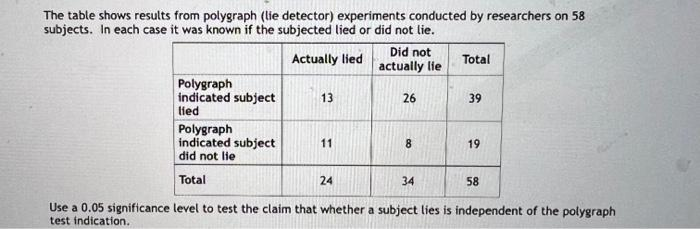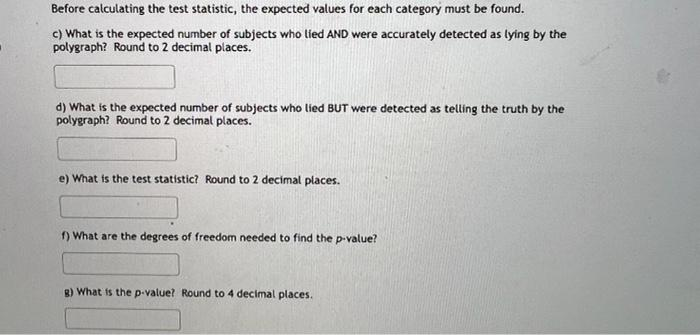Home / Expert Answers / Statistics and Probability / the-table-shows-results-from-polygraph-lie-detector-experiments-conducted-by-researchers-on-58-su-pa519

# (Solved): The table shows results from polygraph (lie detector) experiments conducted by researchers on 58 su ...The table shows results from polygraph (lie detector) experiments conducted by researchers on 58 subjects. In each case it was known if the subjected lied or did not lie. Use a 0.05 significance level to test the claim that whether a subject lies is independent of the polygraph test indication. Before calculating the test statistic, the expected values for each category must be found. c) What is the expected number of subjects who lied AND were accurately detected as lying by the polygraph? Round to 2 decimal places. d) What is the expected number of subjects who lied BUT were detected as telling the truth by the polygraph? Round to 2 decimal places. e) What is the test statistic? Round to 2 decimal places. f) What are the degrees of freedom needed to find the p-value? g) What is the p-value? Round to 4 decimal places.

We have an Answer from Expert

Let's calculate the expected values, test statistic, degrees of freedom, and the p-value for the given data.

To calculate the expected value, we need to assume that lying and the polygraph test indication are independent. The expected value can be calculated using the formula:

It represents the average value we would expect to observe in a given cell of a contingency table if the distribution were purely random. This formula helps estimate the theoretical distribution of values within the table based on the marginal totals.
We have an Answer from Expert